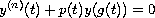Electron. J. Diff. Eqns., Vol. 2005(2005), No. 65, pp. 1-14.

### Asymptotic behaviour of solutions to-order functional differential equations Seshadev Padhi

Abstract:
We establish conditions for the linear differential equationto have property A. Explicit sufficient conditions for the oscillation of the the equation is obtained while dealing with the property A of the equations. A comparison theorem is obtained for the oscillation of the equation with the oscillation of a third order ordinary differential equation.

Submitted September 4, 2004. Published June 23, 2005.
Math Subject Classifications: 34C10, 34K15.
Key Words: Oscillatory solution; nonoscillatory solution; property A.

Show me the PDF file (273K), TEX file, and other files for this article.Seshadev Padhi Department of Applied Mathematics Birla Institute of Technology, Mesra, Ranchi -835 215, India and Department of Mathematics and Statistics Mississippi State University MS-39762, Mississippi State, USA email: ses_2312@yahoo.co.in